# Round Formulas in Excel & Google Sheets

Last updated on September 13, 2023

This tutorial will demonstrate how to use all of Excel and Google Sheets’ rounding functions to round numbers.## Rounding Functions

Excel’s round functions allow you to round numbers to specified number of digits.

### ROUND Function

The ROUND Function rounds numbers to a specified number of digits. When rounding to 0, a number rounds to the nearest whole number. Anything below .5 rounds down and anything equal to or above .5 rounds up.

``=ROUND(B3,C3)``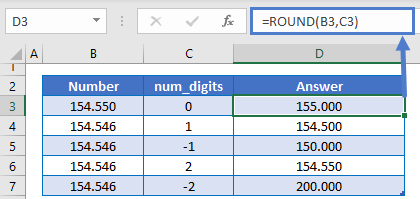As you can see, the ROUND Function takes two inputs:

1. The number to round
2. How many spots to round to the left (negative numbers) or right (positive numbers) of the decimal

### ROUNDUP Function

The ROUNDUP Function works the same as the ROUND Function except it always rounds up to the specified number of digits:

``=ROUNDUP(B3,C3)``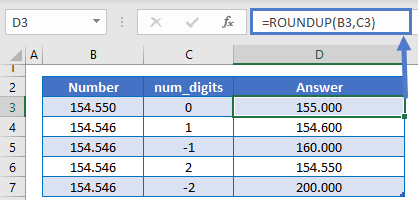### ROUNDDOWN Function

The ROUNDDOWN Function works the same as the ROUND and ROUNDUP Functions except it always rounds down to the specified number of digits:

``=ROUNDDOWN(B3,C3)``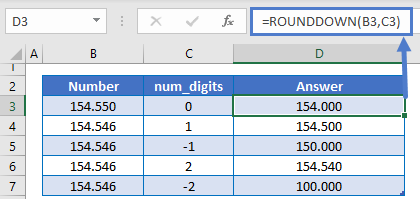### TRUNC Function

The TRUNC Function “truncates” a number after a certain number of digits.

``=TRUNC(B3,C3)``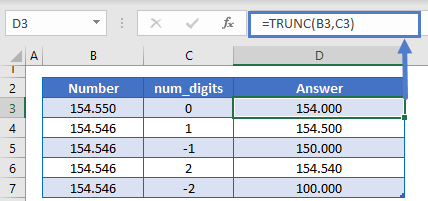### INT Function

The INT Function returns the integer value of a number. It works exactly the same as TRUNC for positive numbers. But will give a different result for negative numbers.

``=INT(B3)``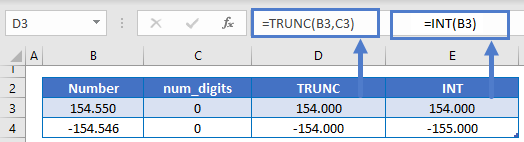### Easy Excel Automation## Rounding Functions – Round to Multiples

The above functions will round to a specified number of digits. To round to a specified multiple use the MROUND, FLOOR, and CEILING Functions.

### MROUND Function

The MROUND Function works similarly to the ROUND Function. However, instead of defining the number of digits to round to, you define the multiple to which to round. Here are some examples:

``=MROUND(B3,C3)``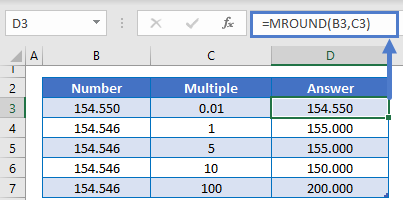### FLOOR Function

The FLOOR Function works just like the MROUND Function, except the FLOOR Function always rounds down.

``=FLOOR(B3,C3)``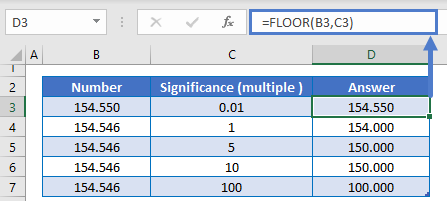### CEILING Function

The CEILING Function works just like the MROUND and FLOOR Functions, except it will always round up.

``=CEILING(B3,C3)``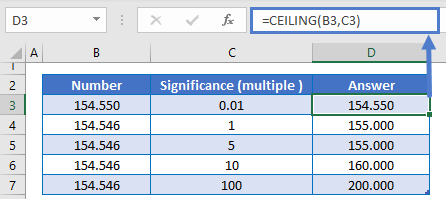## Round Without Formulas

In Excel, you can adjust how numbers are displayed with Number Formatting.  Using Number Formatting you can round how the number is displayed.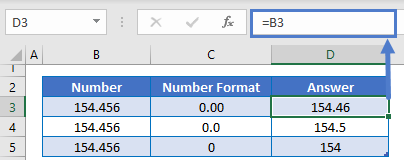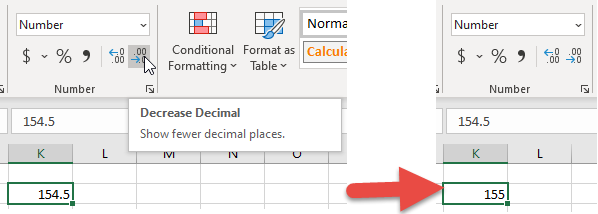## Rounding Examples

### Round Time

With MROUND, you can round time like this:

``=MROUND(B3,"0:15")``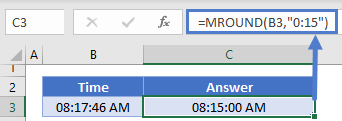### Round Percentages

You can also round percentages with the ROUND Function, just keep in mind that percentages are stored as decimal values, so you must set num_digits accordingly:

``=ROUND(B3,2)``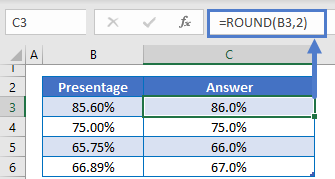AutoMacro | Excel Automation Made Easy | Free Trial

### Round to Nearest Dollar

By setting num_digits to 0, you can round prices to the nearest dollar:

``=ROUND(B3,0)``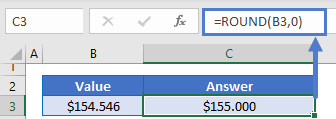### Round to Nearest Cent

Or to the nearest cent with num_digits = 2.

``=ROUND(B3,2)``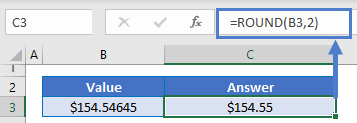### Round Number to n Significant Figures

With some additional calculations, you can use the ROUND Function to round a number to n significant digits:

``=ROUND(A2,B2-(1+INT(LOG10(ABS(A2)))))``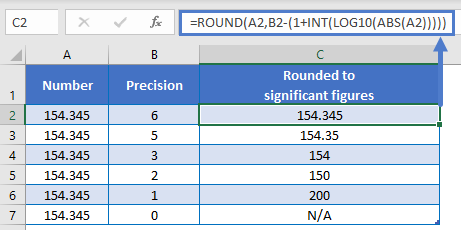### Round a Sum – Round off Formula

You can place other calculations or functions within the ROUND Function. For example, you can round off a decmal:

``=ROUND(SUM(B3:B7),0)``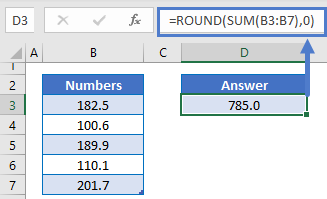AutoMacro | Excel Automation Made Easy | Free Trial

### Round Two Decimal Places

This example will round to two decimal places:

``=ROUND(B3,2)``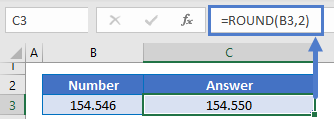### Round to Thousands

This example will round to the thousands place with a negative num_digits:

``=ROUND(B3,-3)``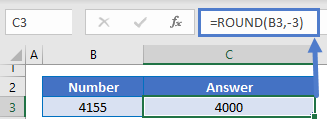### Round Up to Nearest 100

This example will round up to the nearest 100.

``=ROUNDUP(B3,-2)``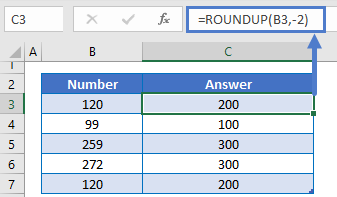### Round to Nearest 5

The MROUND, FLOOR, and CEILING Functions can round to the nearest 5:

``=MROUND(B3,5)``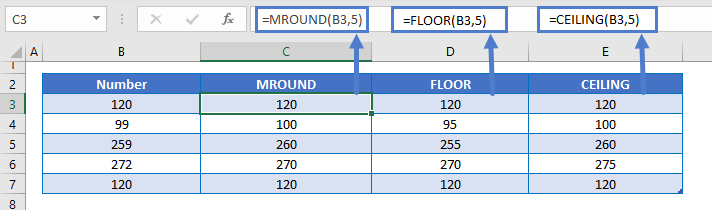AutoMacro | Excel Automation Made Easy | Free Trial

### Calculate Decimal Part of Number

The TRUNC Function can be used to calculate the decimal part of a number.

``=B3-TRUNC(B3,0)``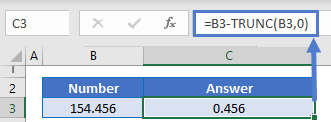This works by first using TRUNC to calculate the integer portion of the number:

``=TRUNC(B3,0)``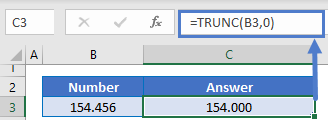## Round Formulas in Google Sheets

All of the above examples work exactly the same in Google Sheets as in Excel.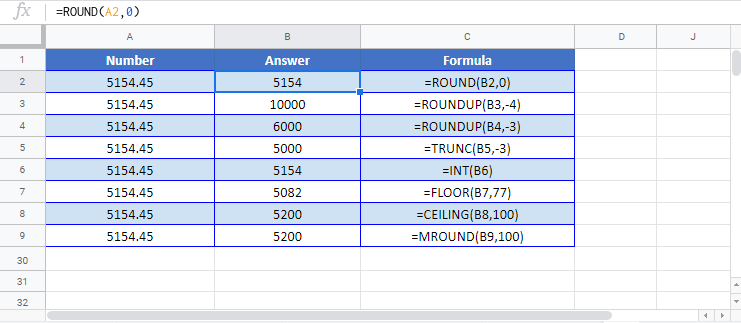### Excel Practice Worksheet

Practice Excel functions and formulas with our 100% free practice worksheets!